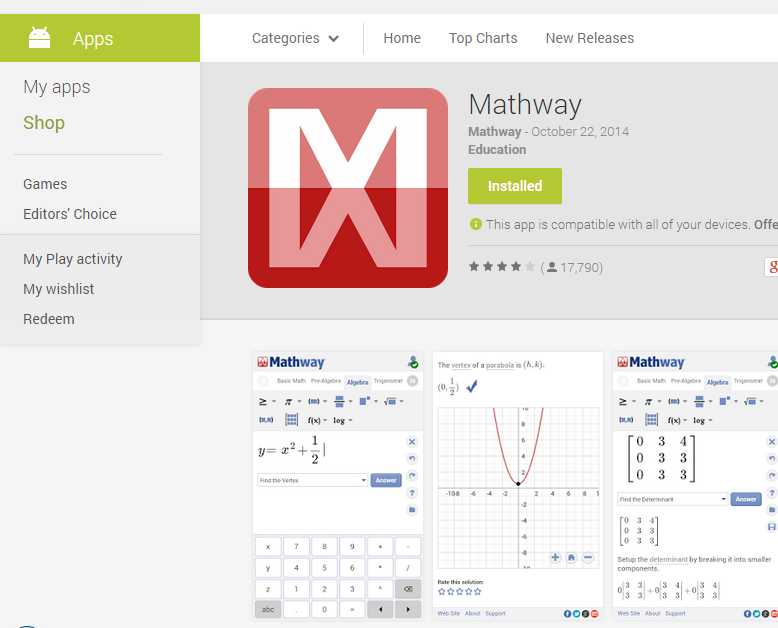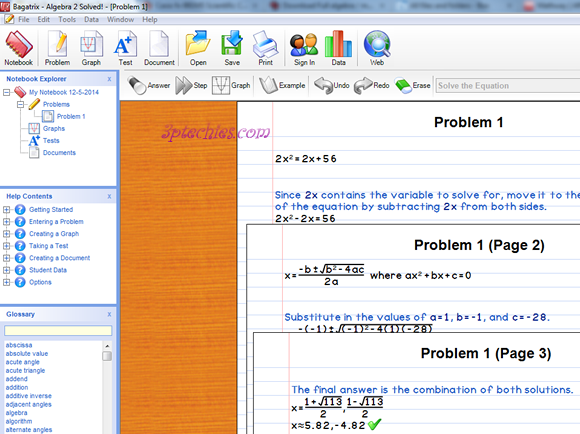# 6 Free Software Apps to Solve Maths and Scientific Equations

6There are free software and applications that can help you solve complicated maths problems and scientific equations, we’ll be looking at the top 6 programs you can use to solve mathematical equations from your computer and/or mobile devices.

### 1. MindMaster

MindMaster is a handy utility app for solving maths and scientific problems. It is a cross-platform app, and as such, available for Windows, Mac, Linux, internet browsers, Google extension, IOS, and Android. Its cloud storage feature allows you to synchronize your data across all your devices.

The Mindmaster science suite has support for online collaboration, formula library, and virtually all commonly used math equation symbols such as relationship and operator, modifier, arrow, separators, fractions, Square roots, Subscripts and superscripts, summation, results and set, integral, underlines and overlines, label arrow, and rectangle.

### 2. College Algebra Solved!

College Algebra Solved! is a student’s friendly software from Bagatrix. It was used by many college students as a tool for attempting maths homework and scientific projects.

It can solve extensive algebraic equations, plot graph, mark trigonometric values as well as present several tests for improving one’s skill in Mathematics and sciences.

Download Link : Bagatrix has stopped the official distribution of this software, luckily, I’ve got a fully functional copy of it in my system which I uploaded here for your convenience.

### 3. Algebra 2 Solved!

Algebra 2 Solved! is an improvement on the College Algebra Solved! software. More functions were added to it, more detailed working methods were used to present solutions to problems.

Solving simultaneous equations, polynomials, surd, and several algebraic equations is made possible, easy, and effortless with the Algebra 2 Solved! PC software.### 4. Mathway [Web & Mobile App]

Mathway combines the power of College Algebra Solved!, Algebra 2 Solved! and Calculus Solved! to bring you an all-efficient web and mobile app to solve complicated mathematical expressions and scientific equations.

Mathway can solve problems in Basic Math, Pre-Algebra, Algebra, Trigonometry, Precalculus, Calculus, Statistics, Finite Math, Linear Algebra, and Chemistry. It is a very handy maths tool for teachers, parents, students, and engineers.

In addition to your Casio fx-991MS Scientific Calculator as an engineer or science student, you should think of getting a working version of Mathway to make your working with maths & equations fun.

Mathway for Mac OS & PC: For windows, mac, browsers and Linux machines, there’s the web-app to help with your mathematics and engineering problems.

### 5. Math Expert

Math Expert is a very useful android app for attempting maths and scientific problems, it is not as robust as the other apps we listed above, but still efficient for engineering and maths students.

### 6. f(x) Mathematics

f(x) Mathematics is a free android app to solve mathematics and scientific problems, it can solve problems related to:

• Statistics
• Differential and integral calculus
• Linear algebra, vectors, matrices
• Complex number calculation
• Modulo calculation with big integer and fractions
• Curve sketching, limes, minima, maxima

f(x) Mathematics calculates any formula you want and show them in a 2d or 3d plot. The natural display shows fractions, roots and exponents as you would expect it from mathematics.

Have you ever used an app or software to attempt a mathematics homework and/or school projects?Obasi Miracle is one of the brains behind 3rd Planet Techies. A webdesign afficionado and IT Support brain-box, he flourishes and revel in helping many-a-techies in their aspiration to become the best they can.

1.ali raza

colculus and snalytical geometry Swokowski chapter 3 edition 6 th

2.John

will bagatrix work on a macbook?

•Obasi Miracle

3.manu sagar
4.bobretti
5.Sharifulrayhan